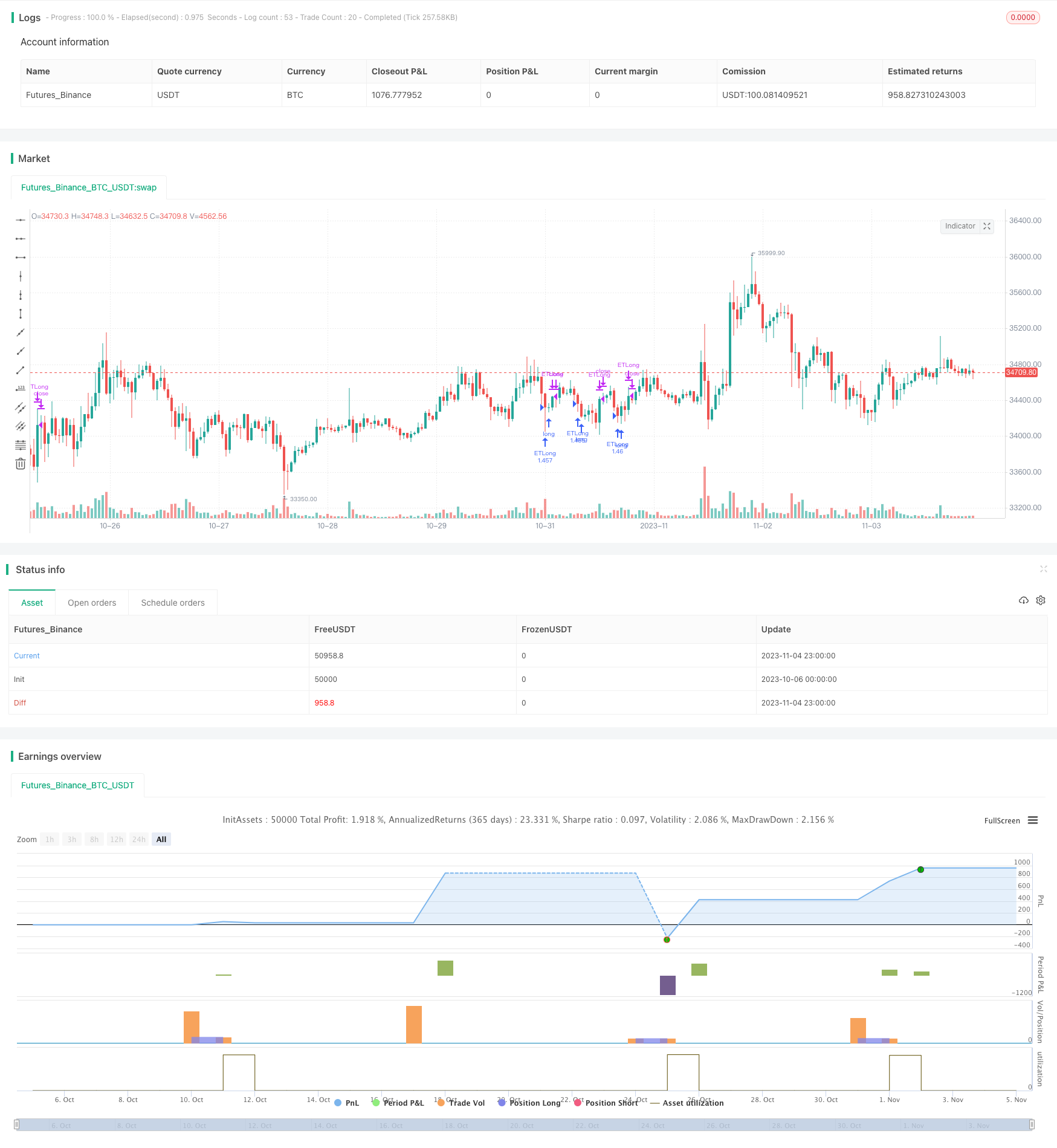# Monday Reversal Intraday Trend Following Strategy

Author: ChaoZhang, Date: 2023-11-06 15:34:06
Tags:## Overview

The main idea of this strategy is to profit from Monday’s intraday reversal using trend following.

## Principles

The core logic is:

1. Check if it is Monday, if yes, continue to next steps;

2. Identify if an uptrend reversal pattern exists - Close < Close and Close < Close;

3. If reversal pattern confirmed, go long at the close of 3rd bar to follow the trend;

4. Exit if today’s high is breached, or stop loss is hit;

5. Close position after 6 hours.

The strategy capitalizes on specific Monday reversal, identifies reversal patterns to go long at relative lows for profits. Stop loss in place to control risks.

1. Profits from Monday reversals during specific periods;

2. Clear entry signals from reversal candlestick patterns;

3. Stop loss and take profit to control risks;

4. Trend following approach maximizes profits;

5. Simple and easy to understand logic;

## Risks

There are some risks:

1. Losses if Monday reversals not significant;

2. Price may retrace after entry leading to stop loss;

3. Sudden market changes may result in large stop loss;

4. Holding too long may also lead to losses;

The solutions are optimizing stop loss, shortening holding time, and controlling single loss size.

## Enhancements

The strategy can be improved by:

1. Using machine learning to identify reversals more accurately;

2. Optimizing stop loss strategies like trailing stop or partial stop loss;

3. Incorporating more factors to judge trend strength;

5. Using algorithms to find optimal parameters;

These can increase the win rate and profitability.

## Conclusion

In conclusion, the strategy capitalizes on Monday reversals, with clear entry/exit rules, to implement a simple trend following strategy. It can achieve better results than fixed stop loss/take profit. Further optimizations are needed to address market uncertainty. The strategy provides a reference for intraday trading.

```/*backtest
start: 2023-10-06 00:00:00
end: 2023-11-05 00:00:00
period: 1h
basePeriod: 15m
exchanges: [{"eid":"Futures_Binance","currency":"BTC_USDT"}]
*/

//@version=4
strategy("ET Forex TurnaroundMonday", overlay=true)
FirstYear = input(2018, minval=2000, maxval=2023, step=1)
FirstMonth = 1 //input(1, minval=1, maxval=12, step=1)
FirstDay = 1 //input(1, minval=1, maxval=31, step=1)

StartHour = input(0)

HoldTime = input(6, step=1)

MM = input(1)

startHour = input(-7, step=1)
endHour = input(34, step=1)
exitHour = input(30, step=1)

startdateCond = (year > FirstYear or (year == FirstYear and (month > FirstMonth or (month == FirstMonth and dayofmonth >= FirstDay))))

iHour = hour
if iHour > 19
iHour := iHour-20
else
iHour := iHour+4

timeCondition = true //(iHour>=startHour and iHour<=endHour and iHour<=exitHour)

since_flat_condition = strategy.position_size == 0

entryPrice=strategy.position_avg_price
EntryLongCondition = dayofweek == (dayofweek.monday+deltaDay) and close < close and close<close and startdateCond //and timeCondition and iHour > StartHour
ExitLongCondition = strategy.position_size > 0  and (close > high)// or close< entryPrice-abs(close-close)*0.2)//(ExitTimeCondition) //iHour >= exitHour or
strategy.initial_capital =50000
// MM Block
lots = if MM < 2
strategy.initial_capital
else
strategy.equity

lots := lots/close

entryPrice:=strategy.position_avg_price
strategy.close("ETLong",when=(ExitLongCondition==true))
strategy.entry("ETLong", strategy.long, qty=lots, comment="OpenLong",when=(EntryLongCondition==true))

```

More